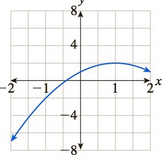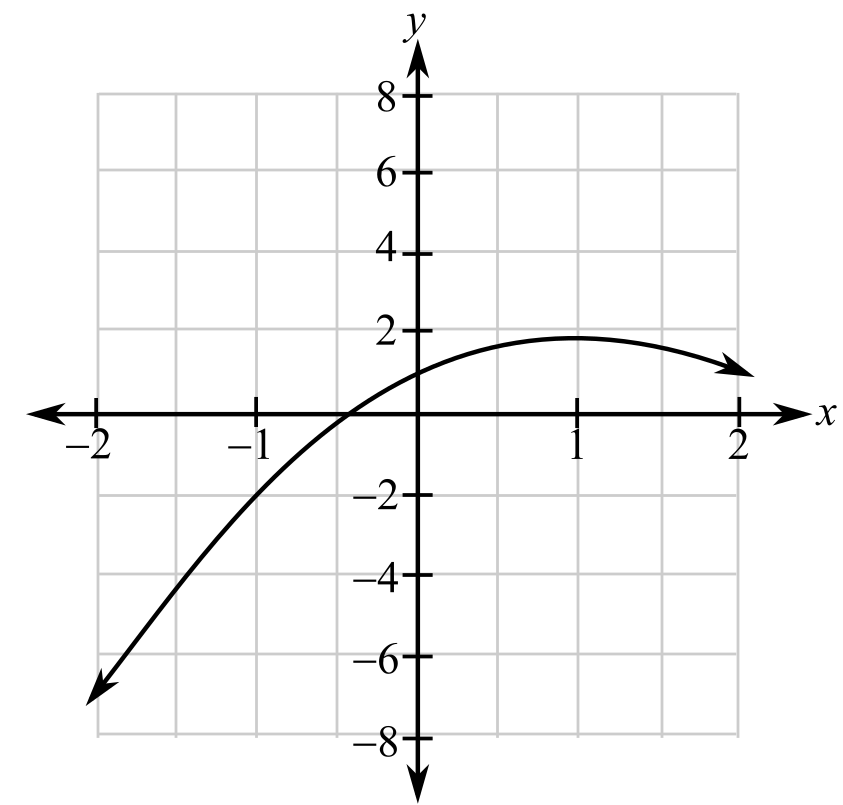Chapter 10.5, Problem 66EFinite Mathematics and Applied Cal...

7th Edition
Stefan Waner + 1 other
ISBN: 9781337274203

Solutions

Chapter
SectionFinite Mathematics and Applied Cal...

7th Edition
Stefan Waner + 1 other
ISBN: 9781337274203
Textbook Problem

In Exercises 65–68 the graph of a function is given. For which x in the range shown is the function increasing? For which x is the function decreasing? [HINT: See Quick Example 6.]To determine

The increasing and decreasing range of the function.

The provided graph is:Explanation

Given information:

The provided graph is:

When the slope of the function is positive then it is increasing function where as if the slope is negative then it is decreasing function.

From the graph one can observe that the curve rebounds at (1,2<

Still sussing out bartleby?

Check out a sample textbook solution.

See a sample solution

The Solution to Your Study Problems

Bartleby provides explanations to thousands of textbook problems written by our experts, many with advanced degrees!

Get Started

In Exercises 2340, find the indicated limit. 40. limx3xx2+72x2x+3

Applied Calculus for the Managerial, Life, and Social Sciences: A Brief Approach

Evaluate the integrals in Problems 1-32.

Mathematical Applications for the Management, Life, and Social Sciences

True or False: f(x) = x2 is decreasing for 10 x 1.

Study Guide for Stewart's Single Variable Calculus: Early Transcendentals, 8th

For 0

Study Guide for Stewart's Multivariable Calculus, 8th